Author: Oscar Cronquist Article last updated on October 13, 2020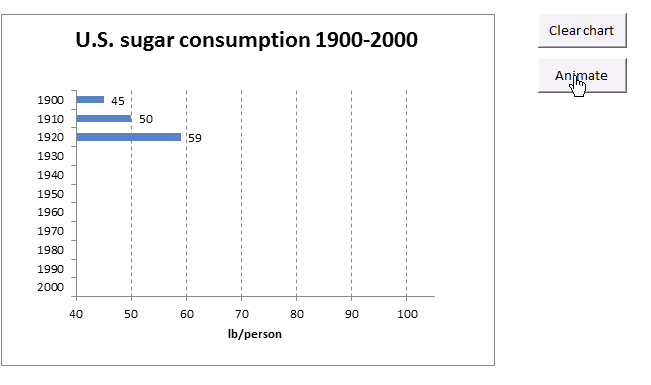This article demonstrates macros that animate bars in a chart bar. The image above shows a bar chart that animates one bar at a time, the bar grows until it reaches the correct value.

The "Clear chart" button clears all values in the chart. The "Animate" button starts the animation. Check out my other animated chart:An animated Excel chart

### Slower animation (Previous Excel versions)

####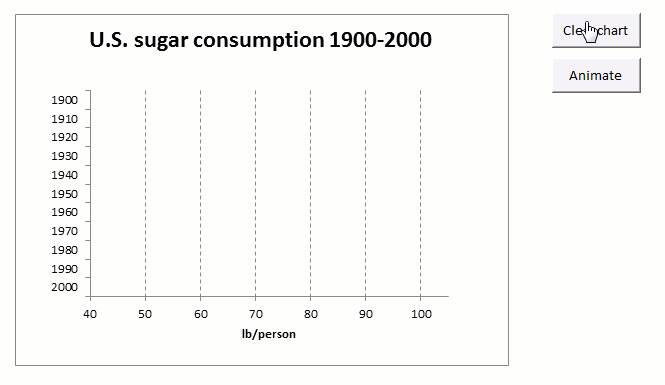The animated image above shows a bar chart animation that has bars that grow more slowly. Press with left mouse button on the "Clear chart" button located to the right of the chart to delete the chart bars.

Press with left mouse button on the "Animate" button located below the "Clear chart" button to start the animation. The "Animate" button has a macro assigned named ClearChart displayed below.

#### VBA Code

```'Name macro
Sub ClearChart()

'Delete values in cell range F3:F13
Range("F3:F13") = ""
End Sub
```
```'Name macro
Sub Animate()

'Dimension variables and declare data types
Dim i As Single, j As Integer, k As Single

'For - next statement, count backwards from 11 to 1 using variable j
For j = 11 To 1 Step -1

'Save value from cell range C3:C13 to variable k based on variable j
k = Range("C3:C13").Cells(j).Value

'For - next statement, count from 40 to number stored in variable k using variable i
For i = 40 To k
'Save value in variable i to cell in cell range F3:F13 based on number stored in variable j
Range("F3:F13").Cells(j) = i

'show changes on screen
DoEvents
Next i
Next j
End Sub
```

### Where to put the VBA code?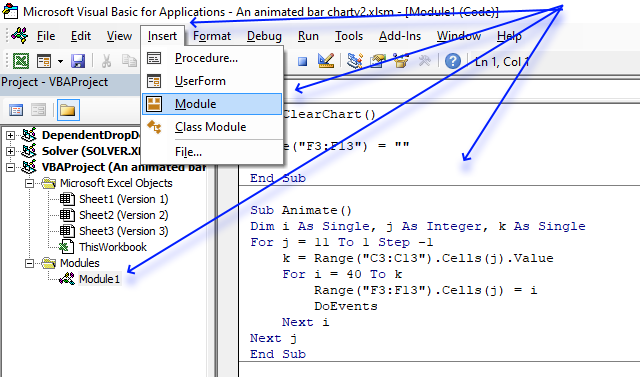You must copy the VBA code and paste it in a module in your workbook before you can use the macros.

1. Copy the VBA code above or below on this webpage.
2. Press Alt + F11 to open the Visual Basic Editor.
3. Press with mouse on the "Insert" on the top menu, see image above.
4. Press with mouse on "Module" to create a module. A module appears in the "Project Explorer" window. This is also shown in the image above.
5. Paste the VBA code to the code module.
6. Go back to Excel.
Note, save the workbook using the file extension *.xlsm (macro-enabled workbook) to keep the VBA code attached to your workbook.

#### Explaining the chart animation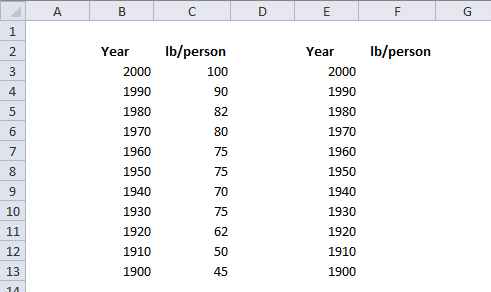The bar chart's data source is cell range E2:F13. The macro begins with cell F13 and starts with value 40.

Then it adds 1 to 40, up to the value in C13 (45). The next cell is F12, starts at 40 and goes up to 50, one by one, and so on. See the picture below. The chart shows these changes in the data source as an animation.

### Slower animation (Excel 365 subscription)

```'Name macro
Sub ClearChart()

'Delete values in cell range F3:F13
Range("F3:F13") = ""
End Sub
```
```'Name macro
Sub Animate()

'Dimension variables and declare data types
Dim i As Single, j As Integer, k As Single

'For - next statement, count backwards from 11 to 1 using variable j
For j = 11 To 1 Step -1

'Save value from cell range C3:C13 to variable k based on variable j
k = Range("C3:C13").Cells(j).Value

'For - next statement, count from 40 to number stored in variable k using variable i
For i = 40 To k
'Save value in variable i to cell in cell range F3:F13 based on number stored in variable j
Range("F3:F13").Cells(j) = i

'show changes on screen
DoEvents

'Repeat to make it work in Excel 365
Next i
Next j
End Sub
```

### A faster animation (Previous Excel versions)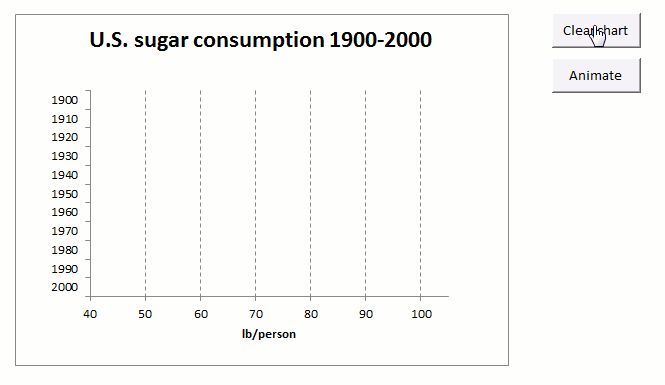The animated image above shows a much faster bar chart animation.

#### VBA Code

```Sub ClearChart()
Range("F3:F13") = ""
End Sub
```
```'Name macro
Sub Animatev2()

'Dimension variables and declare data types
Dim i As Single, j As Integer, k As Single, s As Single

'For ... Next statement. Go from 11 to 1 using variable j
For j = 11 To 1 Step -1

'Save value from cell in cell range C3:C13 to variable k based on variable j
k = Range("C3:C13").Cells(j).Value

'This line makes the animation faster, it calculates how fast the jump between numbers will be
s = (k - 40) / 10

'For ... Next statement. Go from 40 to number saved in variable k using variable i and s
For i = 40 To k Step s

'Save rounded number saved in variable i to cell in cell range F3:F13 based on variable j
Range("F3:F13").Cells(j) = Round(i, 0)

'Show changes on screen
DoEvents
Next i
Next j
End Sub
```

### Faster animation (Excel 365 subscription)

```Sub ClearChart()
Range("F3:F13") = ""
End Sub
```
```'Name macro
Sub Animatev2()

'Dimension variables and declare data types
Dim i As Single, j As Integer, k As Single, s As Single

'For ... Next statement. Go from 11 to 1 using variable j
For j = 11 To 1 Step -1

'Save value from cell in cell range C3:C13 to variable k based on variable j
k = Range("C3:C13").Cells(j).Value

'This line makes the animation faster, it calculates how fast the jump between numbers will be
s = (k - 40) / 10

'For ... Next statement. Go from 40 to number saved in variable k using variable i and s
For i = 40 To k Step s

'Save rounded number saved in variable i to cell in cell range F3:F13 based on variable j
Range("F3:F13").Cells(j) = Round(i, 0)

'Show changes on screen
DoEvents

'Repeat DoEvents to make it work in Excel 365
DoEvents

Next i
Next j
End Sub
```

### Chart Bars (Previous Excel versions)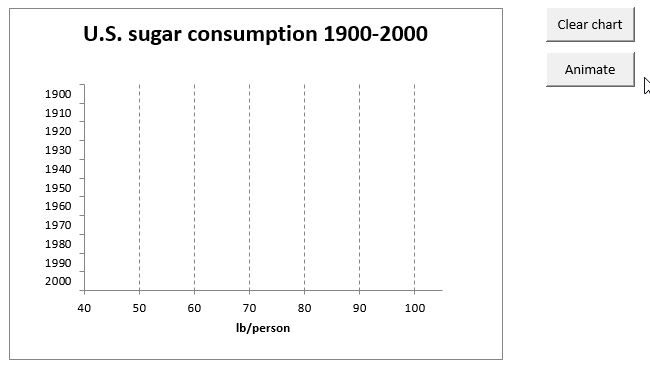```'Name macro
Sub Animatev3()

'Dimension variables and declare data types
Dim i As Single, j As Integer, k As Single, s As Single

For ... Next statement, iterate from 11 to 1
For j = 11 To 1 Step -1

'Save value from cell range C3:C13 based on variable j
k = Range("C3:C13").Cells(j).Value

'Create a small delay using a FOR .. NEXT statement
For i = 1 To 1000

'Refresh screen
DoEvents

Next i

'Save variable k to cell in cell range F3:F13 based on variable j
Range("F3:F13").Cells(j) = k

Next j
End Sub
```

### Chart Bars (Excel 365 subscription)```'Name macro
Sub Animatev3()

'Dimension variables and declare data types
Dim i As Single, j As Integer, k As Single, s As Single

For ... Next statement, iterate from 11 to 1
For j = 11 To 1 Step -1

'Save value from cell range C3:C13 based on variable j
k = Range("C3:C13").Cells(j).Value

'Create a small delay using a FOR .. NEXT statement
For i = 1 To 1000

'Refresh screen
DoEvents

'A second DoEvents is needed to make it work in Excel 365
DoEvents

Next i

'Save variable k to cell in cell range F3:F13 based on variable j
Range("F3:F13").Cells(j) = k

Next j
End Sub
```